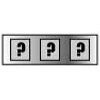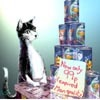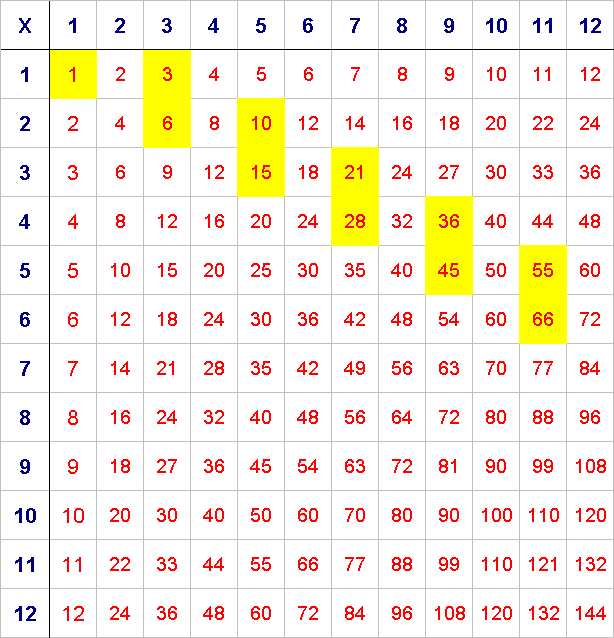#### You may also like### Summing Consecutive Numbers

15 = 7 + 8 and 10 = 1 + 2 + 3 + 4. Can you say which numbers can be expressed as the sum of two or more consecutive integers?### Forgotten Number

I have forgotten the number of the combination of the lock on my briefcase. I did have a method for remembering it...### Human Food

Sam displays cans in 3 triangular stacks. With the same number he could make one large triangular stack or stack them all in a square based pyramid. How many cans are there how were they arranged?

# Triangle Numbers

##### Age 11 to 14Challenge Level

In the multiplication square below, the first eleven triangle numbers $1$, $3$, $6$, $10$, $15$, $21$, $28$, $36$, $45$, $55$, $66$ have been identified.

Can you see a pattern?
Can you predict the positions of the next triangle numbers?
Check to see if you're right.Can you explain why triangle numbers take up these positions in a multiplication square?

Thank you to Paul Stephenson from The Magic Mathworks Travelling Circus who provided the idea for this problem.